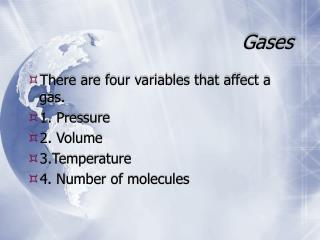DownloadDownload PresentationGases

# Gases

Télécharger la présentation## Gases

- - - - - - - - - - - - - - - - - - - - - - - - - - - E N D - - - - - - - - - - - - - - - - - - - - - - - - - - -
##### Presentation Transcript

1. Gases • There are four variables that affect a gas. • 1. Pressure • 2. Volume • 3.Temperature • 4. Number of molecules

2. The variables • Pressure units - there are many units for pressure. • kPa - kilopascal • Atm - atmospheres • mm Hg - millimeters of Hg • Volume is measured in Liters • Temperature is in Kelvin • K = oC + 273 or oC = K - 273 • If the Kelvin temperature doubles the K.E. doubles.

3. The pressure-volume relationship Boyle’s Law • Pressure and volume are inversely related. • One goes up the other goes down • P1 xV1 = P2 x V2

4. Boyle’s Lawsample problem • A high-altitude balloon contains 30.0 L of helium gas at 103 kPa. What is the volume when the balloon rises to an altitude where the pressure is only 25.0 kPa? • 103 kPa x 30.0 L = 25.0 kPa x V2 • V2 = 124 L

5. Boyle’s Lawsample problem • Your turn • Your birthday balloon travels with you from Lincoln to Denver. The balloons volume is 4.0 L with an atmospheric pressure of 101.3 kPa. You arrive in Denver where the atmospheric pressure is 90.0 kPa. What is the new volume of your balloon?

6. Boyle’s Lawsample problem • Answer • 101.3 kPa x 4.0 L = 90.0 kPa x V2 • V2 = 4.5 L

7. The temperature-volume relationshipCharles’s Law • Volume and temperature have a direct relationship. • One goes up the other goes up • One goes down the other goes down V1= V2 T1 T2

8. Charles’s Lawsample problem • A balloon inflated in a room at 24oC has a volume of 4.00 L. The balloon is then heated to a temperature of 58oC. What is the new volume if the pressure remains constant? 4.00L = V2 297 K 331 K V2 = 4.46 L

9. Charles’s Lawsample problem • Your turn • A balloon is inflated in a room at 24oC and has a volume of 4.00 L. The balloon is placed in a freezer and then removed the volume is now 3.25 L. What was the temperature of the freezer in Celcius?

10. Charles’s Lawsample problem • Answer 4.00 L = 3.25 L 297 K T2 = 241 K which is - 31.7 oC